Math Concepts

# Pick the Odd One Out

140.7k views

 1 Introduction 2 What is 'Odd one out'? 3 Examples 4 Summary 5 Frequently Asked Questions (FAQs)

## Introduction

Picking the “Odd one out” is an activity designed to develop a learner’s observation, application, and analytical skills.

A puzzle (generally images) for the students to find out, with four answer options and in that any three of the options would be similar and the remaining option would be different.

The answer options would vary on the basis of color, shape, size, quantity, category (vegetables, fruits, various types of things, vehicles, animals, and it goes on...)

## Odd One Out

Here is a downloadable pdf that consists of some examples and exercises which may help you understand the concept of Odd one Out better. There is a set of four images/text among which one of them is odd one out. Click on the download button to find them!

## What is 'Odd one out'?

The 'Odd-one-out' is a concept that activates children’s thinking ability around a certain area or subject that is given in a bit of a confusing manner with similar objects or texts.

To correctly identify the odd one out, children need to develop their logical thinking in classifying those objects (or set of options) based on known concepts.

Children need to differentiate the objects and circle the odd one from each set.

This activity has the following pattern:

• Observe the objects
• Identify the common features
• recognize the one with the least common features
• spot that as the odd one out!

The objects can belong to many categories with varying difficulty levels.

To identify the odd one out follows the following pattern:

• Observe the objects carefully
• Describe the objects
• Identify the similarities
• Identify the one which is different or dissimilar

This will help the child develop vocabulary and develop keen observation to identify, classify and analyze.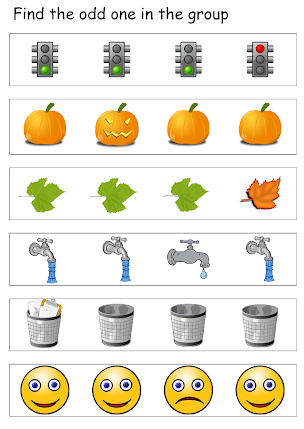Finally, let each child conclude by locating the odd one out and explain their reason behind their solution.

For example, find the odd one out in the following things.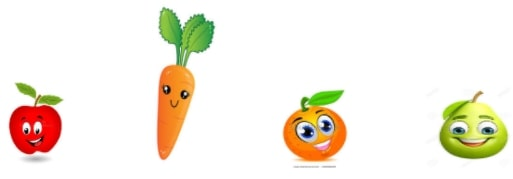Carrot is the ‘odd one out’ as it belongs to the vegetable family. All other options belong to the fruit family.

Let’s see some practical examples to understand better.

1. ### Birthday

Take an example of a classroom of 20 students both boys and girls. One of the students, Vedanth, celebrates his birthday on Aug 7th and so he is not wearing his uniform. Hence he is an ‘Odd One Out’ in that classroom.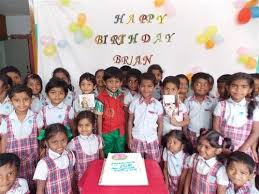Likewise, any birthday boy or birthday girl becomes an ‘odd one out’ on their birthday. If more than one student celebrates a birthday then they all become the odd ones out.

1. ### Ring Finger

Look at this girl”s hands. Which finger is the odd one?

The finger wearing a ring is the ‘odd one out’ among her five right-hand fingers.## Examples

Example: Circle the odd one out in the group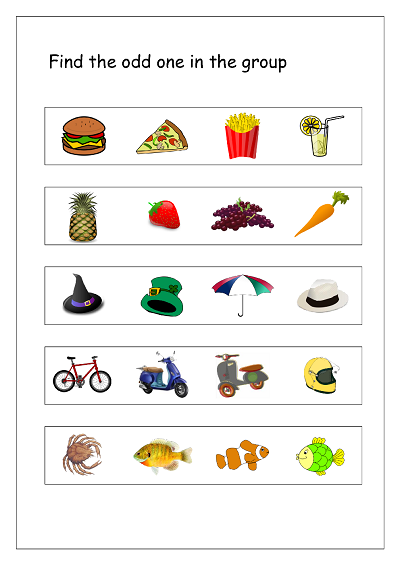1st row - Juice is the odd one out as it is a liquid drink. Other items are snacks and solid ones.

2nd row - Carrot is the odd one out as it belongs to the vegetable family. Other items belong to the fruit family.

3rd row - Umbrella is the odd one out. All other items are hats that are worn on the head. Umbrella is not worn but is held in the hand during extremely sunny or rainy days.

4th row - Helmet is the odd one out. The remaining 3 options are vehicles that are used to drive. Helmets are worn over the head for safety while driving.

5th row - Crab is the odd one out. The other 3 options belong to the fish family.

Example: Pick the odd ones out on the basis of shape, nature, color, size, difference, quantity etc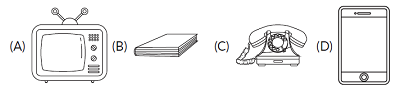(B) Book

Answer: Book is the ‘odd one out’ as it is made up of paper. The other 3 options are electronic means of communication.

Example: Choose the odd number.

(A) 4         (B) 6         (C) 9         (D) 10

 (C) 9

Answer: 9 is the ‘odd one out’ as it is an odd number and 4, 6, and 10 are even numbers.

Example: Choose the odd option.

(A) 9 – 4         (B) 6 – 1        (C) 8 – 3          (D) 7 – 1

 (D) 7-1

Answer: 7-1 is the ‘odd one out’ as the result of subtraction fact 7 - 1 is 6. All the other 3 subtraction facts give a result as number 5.

Example: Choose the odd option.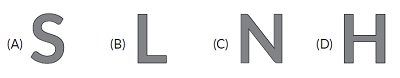(A) S

Answer: ‘S’ is the ‘odd one out’ as it is written using a curved line and the other three letters are written using straight lines.

Example: Circle the odd one out in the group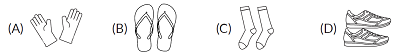(A) Gloves

Answer: Gloves set is the ‘odd one out’ as it is worn on hands. The remaining 3 items are worn in the legs.

Example: Choose the odd option.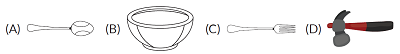(D) Hammer

Answer: Hammer is the ‘odd one out’ as it is a tool that is used for mending. Other options are cutlery items used in the kitchen.

Example: Circle the odd one out in the following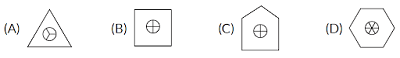(C) Pentagon

Answer: Option C is the ‘odd one out’. In the other 3 images, the number of sides of the image is equal to the number of equal parts drawn inside the circle of the same image. Only in option C, the bigger image [Pentagon] has 5 sides but the circle inside the Pentagon has 4 equal parts.

## Summary

To describe in simple words, something or someone in a group that is different, that does not match with others in a certain logical manner is the odd one out. Practice these questions a lot more, to excel in aptitude exams, competitive exams, etc., to come out with flying colors.

Check the interesting Odd one out games here and learn through fun.

Thanks for spending your time here and in case of any queries or suggestions please do comment below.

Cuemath, a student-friendly mathematics and coding platform, conducts regular Online Live Classes for academics and skill-development, and their Mental Math App, on both iOS and Android, is a one-stop solution for kids to develop multiple skills. Understand the Cuemath Fee structure and sign up for a free trial.